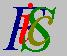Mauro Murzi's pages on Philosophy of Science - Quantum mechanics
The effect of measurement on Ψ functionNon commutative operators Average values# [5. Heisenberg indeterminacy principle.]

## The effect of measurement on Ψ function.

The state of a quantum system is described by a function Ψ(q) that can be represented as a sum of the eigenfunctions of a physical quantity u:where the square of the module of the expansion coefficients σi determines the probability that u has the value ui .

Let u and v two non commutative physical quantities; they have two different sets of eigenfunctions. Suppose that a measurement of u is performed, and the resulting value for u is uk . Thus the probability that u has the value uk is equal to 1. Hence σk = 1 and σi = 0 for every i≠k. The equation (5.2) becomes Ψ u(q) = φku(q)  (the superscript u in the equation marks the fact that the state of the system is determined immediately after a measurement of u).

Immediately after the measurement of u, a measurement of v is performed; the result is vh and thus equation (5.2) becomes Ψ v(q) = φhv(q) . Since u and v have different sets of eigenfunctions, we have φku(q) ≠ φhv(q) and thus Ψ u(q) ≠ Ψ v(q) . A measurement of v, performed after the measurement of a non commutative quantity u, changes the function describing the state of the system.

The measurement of a quantity determines a function Ψ describing the quantum system. If, immediately after this measurement, another one is performed on a non commutative quantity, a different function Ψ is determined. Since function Ψ completely describes the status of the system, we must conclude that the second measurement has altered the state of the system. The situation is different if we consider two commutative entities, since they have the same set of eigenfunctions.

We can summarize the situation in the following way: In a quantum system, described by a function Ψ defined as the sum of the eigenfunctions of an entity, the measurement of a commutative entity does not alter the state of the system, while the measurement of a non commutative entity does alter the state of the system.

A consequence of the fact that two non commutative entities have different sets of eigenfunctions is that there is no functions Ψ which, as the same time, can be written as the sum of the eigenfunctions of two non commutative entities. Thus there is no physical state corresponding to a quantum system in which two commutative entities have, at the same time, definite unique values. If u and v are non commutative entities, then there is no quantum system in which u(t) = uo and v(t) = vo for the same time t. As an example, consider a quantum particle moving along the x-axis; there is no physical state of this particle corresponding to the situation in which the particle has a definite position and definite momentum in the same time. As an obvious consequence, it is impossible to measure the exact position and momentum of a quantum particle, since there is no physical state corresponding to such measurement: There is nothing such as an exact position and momentum of a quantum particle. The impossibility of a such kind of measurement is not a human limitation, but it is a limitation of the applicability of classical concepts, which assign a definite position and momentum to a particle. In quantum mechanics the concept of the path of a particle is not applicable; it is only a classical notion, used as a first approximation, that in some circumstances is inadequate.Non commutative operators Average values